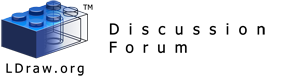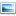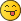Calculate Angles (in LDCad) - Printable Version +- LDraw.org Discussion Forums (https://forums.ldraw.org) +-- Forum: LDraw Programs (https://forums.ldraw.org/forum-7.html) +--- Forum: LDraw Editors and Viewers (https://forums.ldraw.org/forum-11.html) +--- Thread: Calculate Angles (in LDCad) (/thread-22169.html) Calculate Angles (in LDCad) - Jaco van der Molen - 2017-05-12 Hi all, Is there a way to exactly calculate the angles the 1x5 beam and 1x10 brick make? I kind of know how to do this in LDCad, but with these I don't know how. I have done this by hand and eye, but I'd like it to be exact.CalculateAngles.png (Size: 25.41 KB / Downloads: 219) Thanks! RE: Calculate Angles (in LDCad) - Jaco van der Molen - 2017-05-12 Never mind, I got it. Suddenly remembered how to show info and work from 90 degree angles with a "helper" pin.The only thing that I do not fully understand is the sequence to select the pins. Selecting like I did I found the angles of the red triangle to be the ones I needed :-) RE: Calculate Angles (in LDCad) - Philippe Hurbain - 2017-05-12 Method to close a triangle in LDCad: Select one "end" pin, then the matching articulation pin, then the other articulation pin and finally the last "end" pin. Right click -> selection info -> display the information panel and triangle angles. Choose either the red or green lines configuration depending on your needs. Click on the box with matching outline color to copy values in clipboard. Now select first articulation pin (to define rotation center) and parts belonging to first triangle arm. Press Ctlr R to enter manual rotation, and paste clipboard in angle value. Eliminate the second value, as we are working with the first arm. Accept. Select second articulation pin (to define rotation center for the second arm) and parts belonging to second triangle arm. Press Ctlr R to enter manual rotation, and paste clipboard in angle value. Eliminate the first value, as we are working with the second arm. Accept. Get rid of extraneous "end" pin(s). ...done! RE: Calculate Angles (in LDCad) - Jaco van der Molen - 2017-05-12 (2017-05-12, 15:17)Philippe Hurbain Wrote: Method to close a triangle in LDCad: Select one "end" pin, then the matching articulation pin, then the other articulation pin and finally the last "end" pin. Right click -> selection info -> display the information panel and triangle angles. Choose either the red or green lines configuration depending on your needs. Click on the box with matching outline color to copy values in clipboard. Now select first articulation pin (to define rotation center) and parts belonging to first triangle arm. Press Ctlr R to enter manual rotation, and paste clipboard in angle value. Eliminate the second value, as we are working with the first arm. Accept. Select second articulation pin (to define rotation center for the second arm) and parts belonging to second triangle arm. Press Ctlr R to enter manual rotation, and paste clipboard in angle value. Eliminate the first value, as we are working with the second arm. Accept. Get rid of extraneous "end" pin(s). ...done! Thanks Philo for those steps. My problem was only step one. I just go by much trial and error to find the right pins and select sequence. The way you put it I understand! Thanks. The rest I knew, but just forgot. RE: Calculate Angles (in LDCad) - N. W. Perry - 2019-10-30 There's another way to do this, by finding the intersection of two circles. This might be handy if you need to do it in a program that doesn't have LDCad's triangle calculation feature (or if, as I did, you forgot that feature exists and spent an hour figuring it out for yourself and are feeling all clever about it).To illustrate this, I whipped up the same model in LDCad. I've added some circular paths to show the circles, but you actually don't need them. All you have to do now is calculate the intersection of the two circles (highlighted in yellow).It turns out that's pretty simple math (for a computer) if you know the X,Y position of the center of each circle (we do: the selected pins) and its radius (we can find out: by using LDCad's selection info, or in this case just counting studs). You can look up the mathematical formula if you want, but it's much quicker to use an online calculator—I found this one, which works perfectly. So let's enter the values we know: we need the X and Y coordinates of the selected pins (we can ignore the Z), and the radii (distance to the end pins: 140 and 80)And the result, complete with a little graph of the two circles:(Note that the graph is a mirror image of the circles in our project, because of the opposite orientation of LDraw's Y axis. But the numbers themselves are correct.) Of the two intersections found, choose the one that you want to use—in this case it's the second one. Create a new helper part and place it at those X,Y coordinates, then use Selection Info (okay, I guess you still need LDCad for this…) to find the rotation angle you need:Repeat for the other beam, apply the rotations to both parts, and you're done!RE: Calculate Angles (in LDCad) - Jaco van der Molen - 2019-10-30 (2019-10-30, 2:48)N. W. Perry Wrote: There's another way to do this, by finding the intersection of two circles. This might be handy if you need to do it in a program that doesn't have LDCad's triangle calculation feature (or if, as I did, you forgot that feature exists and spent an hour figuring it out for yourself and are feeling all clever about it).To illustrate this, I whipped up the same model in LDCad. I've added some circular paths to show the circles, but you actually don't need them. All you have to do now is calculate the intersection of the two circles (highlighted in yellow). It turns out that's pretty simple math (for a computer) if you know the X,Y position of the center of each circle (we do: the selected pins) and its radius (we can find out: by using LDCad's selection info, or in this case just counting studs). You can look up the mathematical formula if you want, but it's much quicker to use an online calculator—I found this one, which works perfectly. So let's enter the values we know: we need the X and Y coordinates of the selected pins (we can ignore the Z), and the radii (distance to the end pins: 140 and 80) And the result, complete with a little graph of the two circles: (Note that the graph is a mirror image of the circles in our project, because of the opposite orientation of LDraw's Y axis. But the numbers themselves are correct.) Of the two intersections found, choose the one that you want to use—in this case it's the second one. Create a new helper part and place it at those X,Y coordinates, then use Selection Info (okay, I guess you still need LDCad for this…) to find the rotation angle you need: Repeat for the other beam, apply the rotations to both parts, and you're done! Nice! RE: Calculate Angles (in LDCad) - Orion Pobursky - 2019-10-30 I have a raft of helper spreadsheets for exactly this (circle-circle, circle-line, etc...): https://docs.google.com/spreadsheets/d/1UKP13-fEiShvChxOy1pCrlV4W_47rUEnwV7xOgUQWBc/edit?usp=sharing RE: Calculate Angles (in LDCad) - Roland Melkert - 2019-10-30 (2019-10-30, 2:48)N. W. Perry Wrote: There's another way to do this, by finding the intersection of two circles. This might be handy if you need to do it in a program that doesn't have LDCad's triangle calculation feature (or if, as I did, you forgot that feature exists and spent an hour figuring it out for yourself and are feeling all clever about it).I setup the selection info stuff before the scripting existed and didn't want to force a certain way to apply the angles etc. But you could script your method, and apply the angles in one go if wanted. FYI: This is what the selection info does internally: Code:```        const double a=relPnt.length(relPnt); const double b=relPnt.length(relPnt); const double c=relPnt.length(relPnt); const double angAB=rad2Deg(acos((a*a+b*b-c*c)/(2*a*b))); const double angBC=rad2Deg(acos((b*b+c*c-a*a)/(2*b*c))); TGLVector3d BADir=relPnt-relPnt; BADir.normalize(); TGLVector3d BCDir=relPnt-relPnt; BCDir.normalize(); TGLVector3d CBDir=BCDir*-1.0; TGLVector3d CDDir=relPnt-relPnt; CDDir.normalize(); TGLVector3d planeNor(true); planeNor.comp[sCompIdx]=1.0; const double intAngAB=rad2Deg(planeNor.calcSignedAngleBetween(BCDir, BADir)); const double intAngBC=rad2Deg(planeNor.calcSignedAngleBetween(CBDir, CDDir)); const double angABCor=intAngAB+angAB; const double angBCCor=intAngBC-angBC; const double angABCorInv=intAngAB-angAB; const double angBCCorInv=intAngBC+angBC;``` RE: Calculate Angles (in LDCad) - N. W. Perry - 2019-10-30 (2019-10-30, 19:18)Roland Melkert Wrote: I setup the selection info stuff before the scripting existed and didn't want to force a certain way to apply the angles etc. But you could script your method, and apply the angles in one go if wanted. Yes, it was pretty easy to find the formula for circle intersections as a code snippet, too. I bet it would be pretty easy to incorporate into an editor, for those with a little more experience in that than I have. :-) This also makes me wonder, could the circles method be used for any situation that the triangle method can't? Or is it really just a different way of doing the same exact thing? RE: Calculate Angles (in LDCad) - Philippe Hurbain - 2019-10-31 (2019-10-30, 2:48)N. W. Perry Wrote: There's another way to do this, by finding the intersection of two circles. This might be handy if you need to do it in a program that doesn't have LDCad's triangle calculation featureThough it also needs to have the possibility to set precise coordinates or turn by a precise angle, two possibilities that AFAIK are not available eg. in LDD or Studio... RE: Calculate Angles (in LDCad) - N. W. Perry - 2019-11-01 (2019-10-31, 10:50)Philippe Hurbain Wrote: Though it also needs to have the possibility to set precise coordinates or turn by a precise angle, two possibilities that AFAIK are not available eg. in LDD or Studio... Definitely a major shortcoming of Studio, and one that I've suggested they incorporate into future versions.